# Integers + subtracting - examples

1. Unknown xIf we add to unknown number 21, then divide by 6 and then subtract 51, we get back an unknown number. What is this unknown number?
2. MistakeNicol mistake when calculate in school. Instead of add number 20 subtract it. What is the difference between the result and the right result?
3. Opposite numbers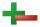Calculate opposite numbers (additive inverse) to given ones:
4. Thermometer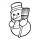The thermometer showed -3°C at morning. Then temperature was increased by 1°C again increased by 1°C and then decreased by 1°C and then decreased by 4°C. Which terminal temperature thermometer shows?
5. Integers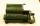May be the sum of two integers less than their difference?
6. Numbers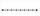Find number on thenumber line that has same distance from this numbers:
7. With bracket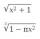Calculate (evaluate) simple mathematical expression with a negative numbers and a bracket: 13+15*5-2*(-6)
8. Freezer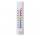Temperature is -8 degrees Celsius in the freezer. Outside temperature is 2 degrees Celsius. One minute took to change temperature by one degree Celsius if we open door. How many minutes the temperature in the freezer will rise?
9. Expression 6Evaluate expression: -6-2(4-8)-9
10. Brackets 2Add parenthesis to make true: 5-2×6-4+2=5
11. Degrees 2The temperature was 3°F and falls four degrees Fahrenheit. What is actually temperature?
12. The temperatureThe temperature at 1:00 was 10 F. Between 1:00 and 2:00, the temperature dropped 15F. Between 2:00 and 3:00, the temperature rose 3F. What is the temperature at 3:00?In about 12 hours in North Dakota the temperature rose from -33 degrees farenheit to 50 degrees farenheit. By how much did the temperature change?